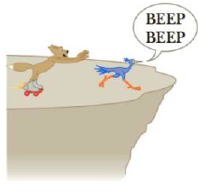Chapter 3, Problem 59AP

Chapter
Section
Textbook Problem

The determined Wile E. Coyote is out once more to try to capture the elusive roadrunner. The coyote wears a new pair of power roller skates, which provide a constant horizontal acceleration of 15.0 m/s2, as shown in Figure P3.59. The coyote starts off at rest 70.0 m from the edge of a cliff at the instant the roadrunner zips in the direction of the cliff, (a) If the roadrunner moves with constant speed, find the minimum speed the roadrunner must have to reach the cliff before the coyote. (b) If the cliff is 1.00 × 102 m above the base of a canyon, find where the coyote lands in the canyon. (Assume his skates are still in operation when he is in “flight” and that his horizontal component of acceleration remains constant at 15.0 m/s2.)Figure P3.59

(a)

To determine
The minimum speed the roadrunner must have to reach the cliff before the cayote.

Explanation

The displacement in the horizontal direction is,

Δx=v0xt+12axt2

Here,

v0x is the horizontal component of the initial velocity

t is the time taken

ax is the horizontal acceleration

Substitute 70.0m for Δx , 15.0m/s2 for ay and 0m/s for v0y to find t .

70.0m=(0m/s)t+12(15.0m/s2)t2t=2(70

(b)

To determine
Where the coyote lands in the canyon.

Still sussing out bartleby?

Check out a sample textbook solution.

See a sample solution

The Solution to Your Study Problems

Bartleby provides explanations to thousands of textbook problems written by our experts, many with advanced degrees!

Get Started

Find more solutions based on key concepts